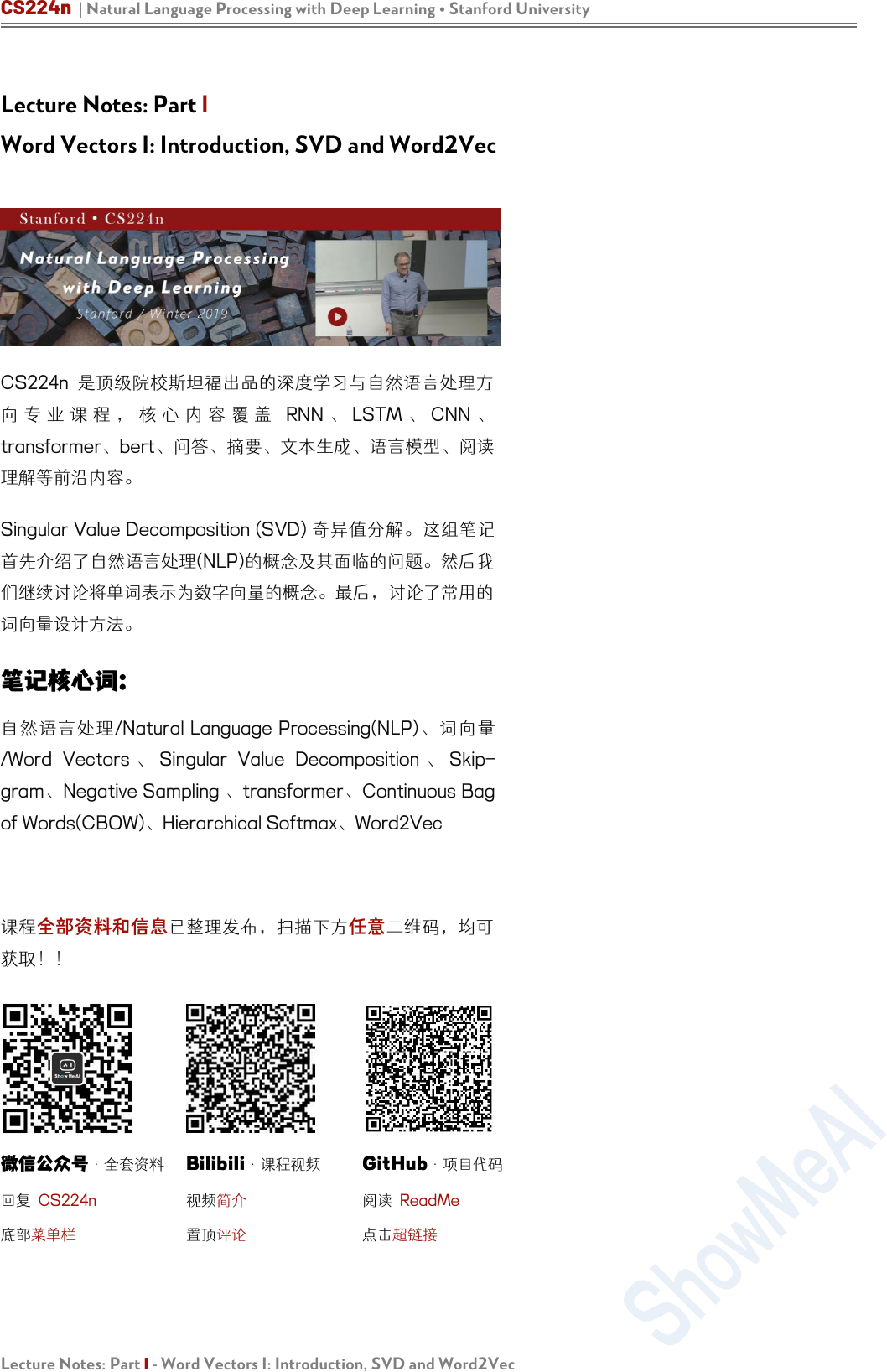CS224n | Natural Language Processing with Deep Learning Stanford University
Lecture Notes: Part I - Word Vectors I: Introduction, SVD and Word2Vec
Lecture Notes: Part I
Word Vectors I: Introduction, SVD and Word2Vec
CS224n
RNN LSTM CNN
transformerbert问答要、成、模型

Singular Value Decomposition (SVD)

/Natural Language Processing(NLP)
/Word Vectors Singular Value Decomposition Skip-
gramNegative Sampling transformerContinuous Bag
of Words(CBOW)Hierarchical SoftmaxWord2Vec

Bilibili·课程视频

GitHub·项目代码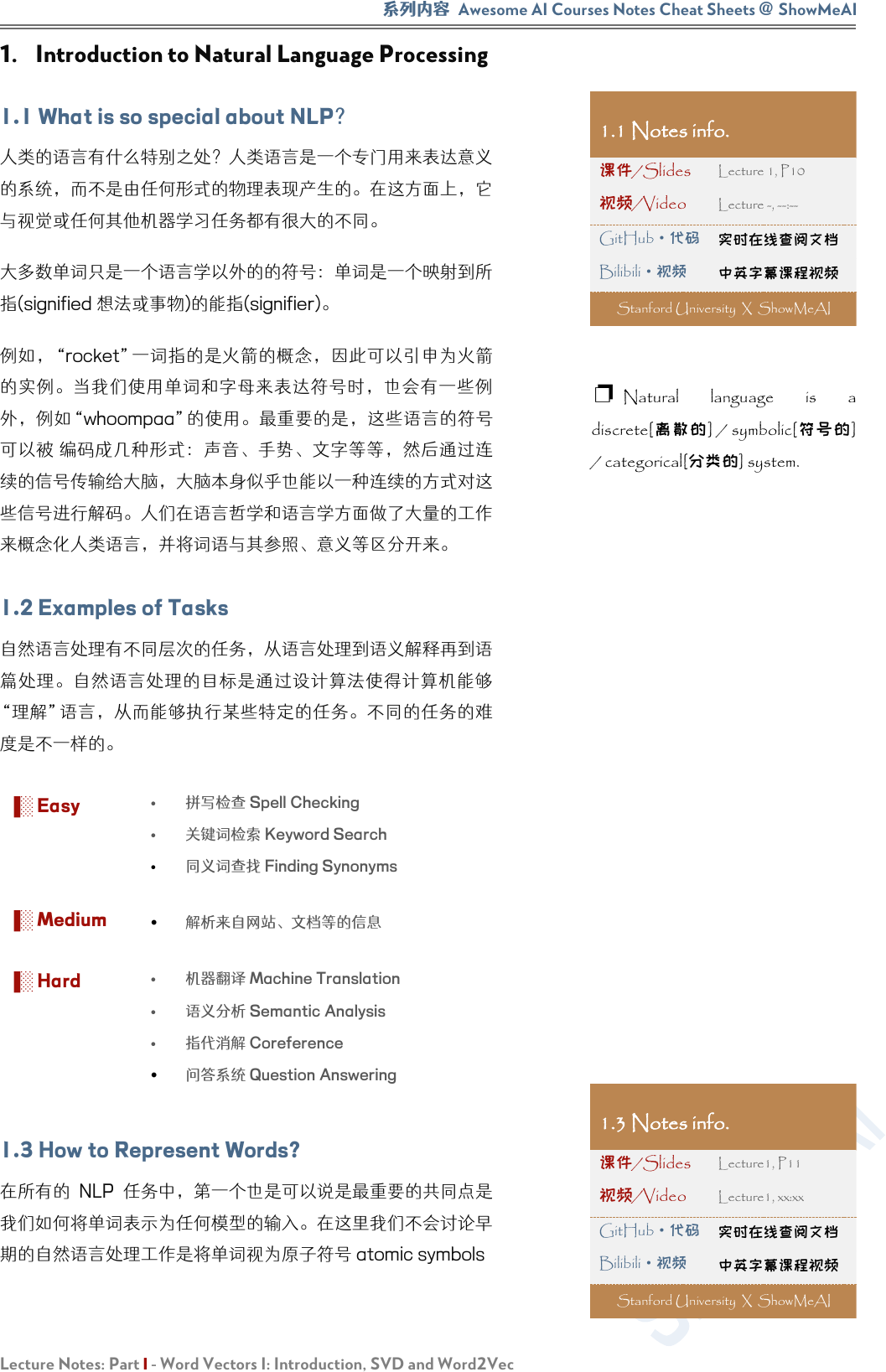Awesome AI Courses Notes Cheat Sheets
@
ShowMeAI
Lecture Notes: Part I - Word Vectors I: Introduction, SVD and Word2Vec
1. Introduction to Natural Language Processing
1.1 What is so special about NLP

(signified 想法或事)的能(signifier)
“rocket”是火

whoompaa使

1.2 Examples of Tasks

▐░ Easy

▐░ Medium

▐░ Hard

1.3 How to Represent Words?

1.1 Notes info.

GitHub·代码
Bilibili
·视频
Stanford University X ShowMeAI
Natural language is a
discrete[] / symbolic[]
/ categorical[分类的] system.
1.3 Notes info.

GitHub·代码
Bilibili·视频
Stanford University X ShowMeAI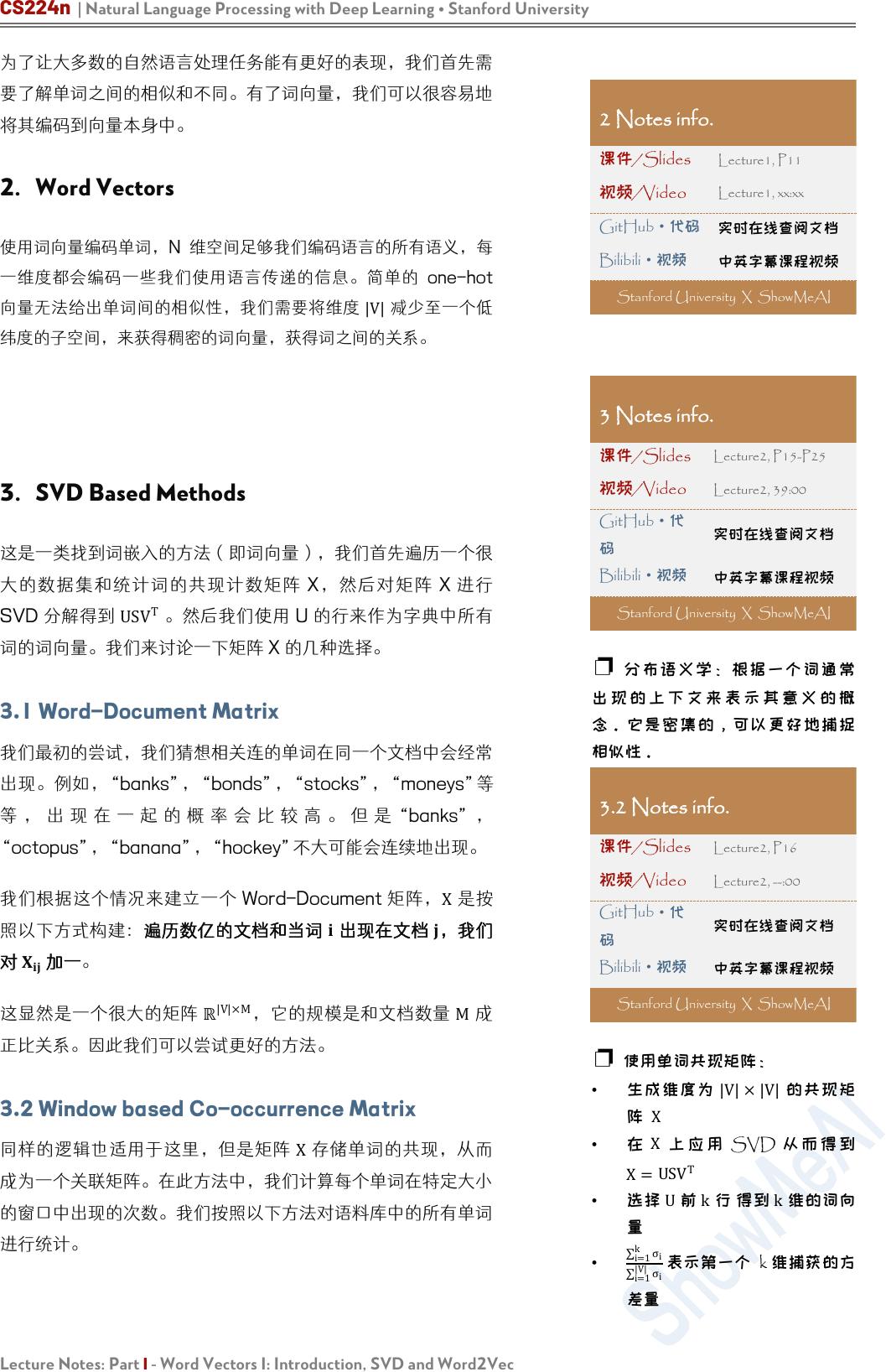CS224n | Natural Language Processing with Deep Learning Stanford University
Lecture Notes: Part I - Word Vectors I: Introduction, SVD and Word2Vec

2. Word Vectors

|V|

3. SVD Based Methods

X X
SVD US
V
T

3.1 Word-Document Matrix

“banks”“bondsstocks”“moneys
“banks”
“octopus”“banana”“hockey不大可能会连续地出现
Word-Document
X

，我


|V|×M
M

3.2 Window based Co-occurrence Matrix
X

2 Notes info.

GitHub
·代码
Bilibili·视频
Stanford University X ShowMeAI
3 Notes info.

Lecture2, P15-P25

Lecture2, 39:00
GitHub·代

Bilibili·视频

Stanford University X ShowMeAI

3.2 Notes info.

Lecture2, P16

Lecture2, --:00
GitHub
·代

Bilibili·视频

Stanford University X ShowMeAI

|V|×|V|
X
X
SVD
X=
USV
T
U
k
k
i=1
k
σ
i
i=1
|V|
σ
i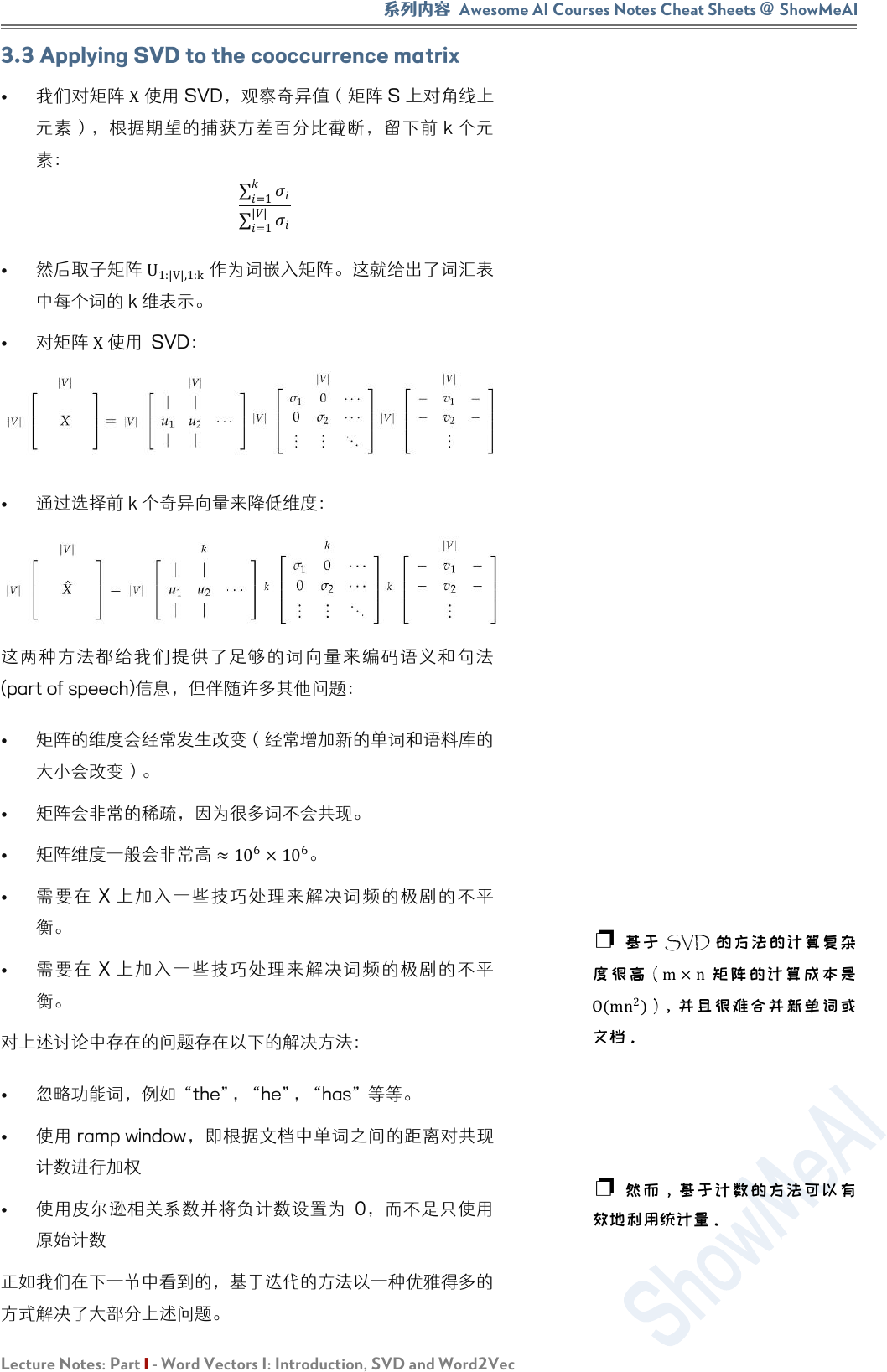Awesome AI Courses Notes Cheat Sheets
@
ShowMeAI
Lecture Notes: Part I - Word Vectors I: Introduction, SVD and Word2Vec
3.3 Applying SVD to the cooccurrence matrix

X

k

=1
=1
||

U
1:|V|,1:k

(part of speech)信息，但伴随许多其他问题：

6
×10
6
X

X

SVD
(
m×n
O(
mn
2
)
)CS224n | Natural Language Processing with Deep Learning Stanford University
Lecture Notes: Part I - Word Vectors I: Introduction, SVD and Word2Vec
4. Iteration Based Methods - Word2vec

(亿

1986

2011] 设计的模型首先将每个单词转换为向量。对每个特定的任

Word2vec
Word2vec 是一个软件包实际上包含：
continuous bag-of-words CBOW
skip-gramCBOW
skip-gram

negative sampling hierarchical
softmaxNegative sampling

4.1 Language Models (Unigrams, Bigrams, etc.)

“The cat jumped over the puddle”

“stock boil fish is
toy”

4 Notes info.

Lecture1, P19

Lecture1, 42:00
GitHub·代

Bilibili·视频

Stanford University X ShowMeAI
SVD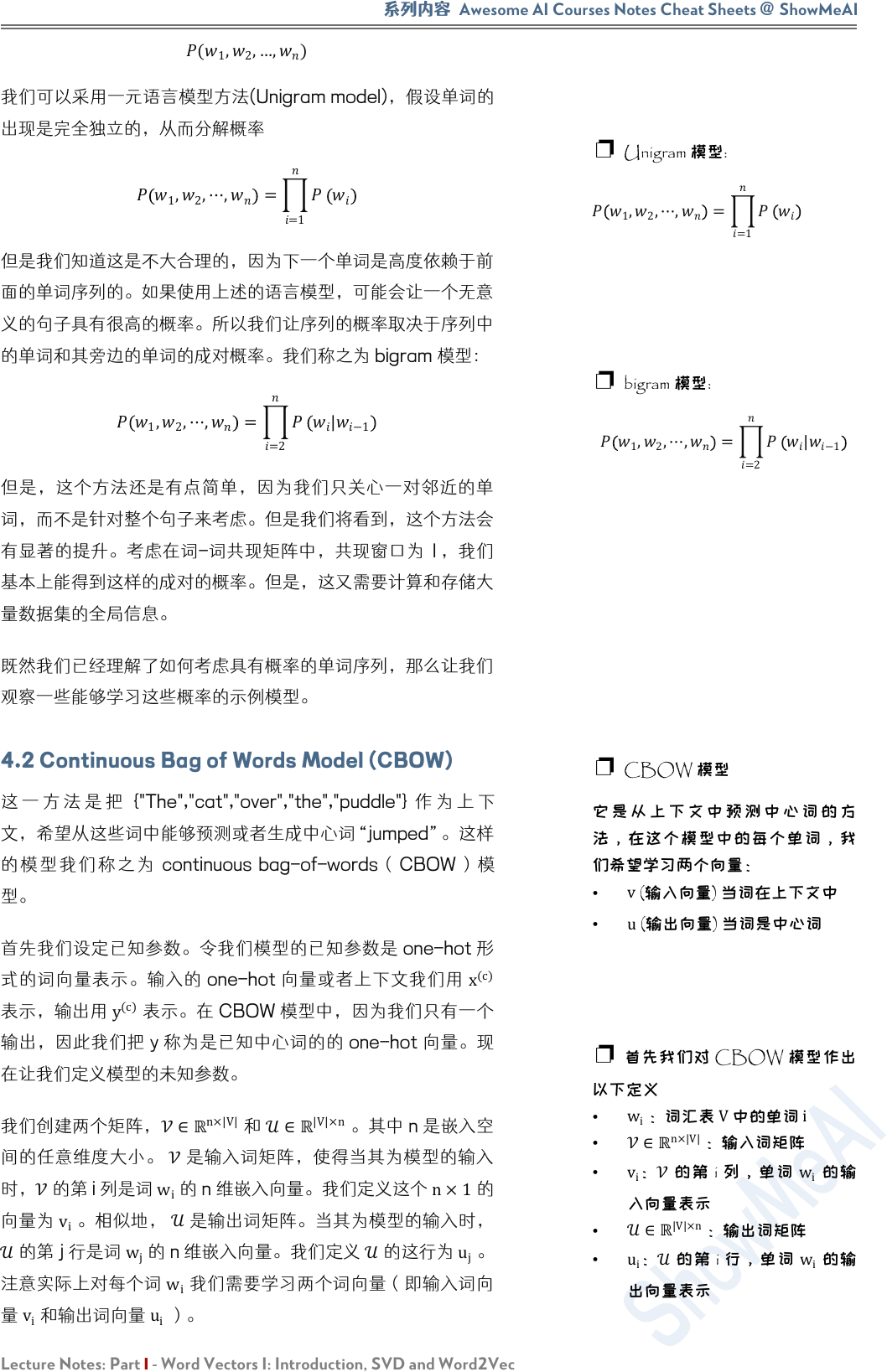Awesome AI Courses Notes Cheat Sheets
@
ShowMeAI
Lecture Notes: Part I - Word Vectors I: Introduction, SVD and Word2Vec
(
1
,
2
,…,
)

1
,
2
,⋯,
=
=1

1
,
2
,⋯,
=
=2
|
−1

4.2 Continuous Bag of Words Model (CBOW)
{"The","cat","over","the","puddle"}

continuous bag-of-words CBOW

one-hot
x
(c)

(c)

y one-hot 。现

n×|V|
|V|×n
。其 n 嵌入

i
n 维嵌入向量。我们定义这个 n×1

i
。相

w
j
n 嵌入向量。我们定义

u
j

w
i

v
i

u
i
）。
Unigram 模型:
1
,
2
,⋯,
=
=1
bigram 模型:
1
,
2
,,
=
=2
|
−1
CBOW 模型

v
(输入向量) 当词在上下文中
u
(输出向量) 当词是中心
CBOW

w
i
：词汇表
V

i
n×|V|
：输入词矩
v
i
i
w
i

|V|×n
：输出词矩
u
i
i
w
i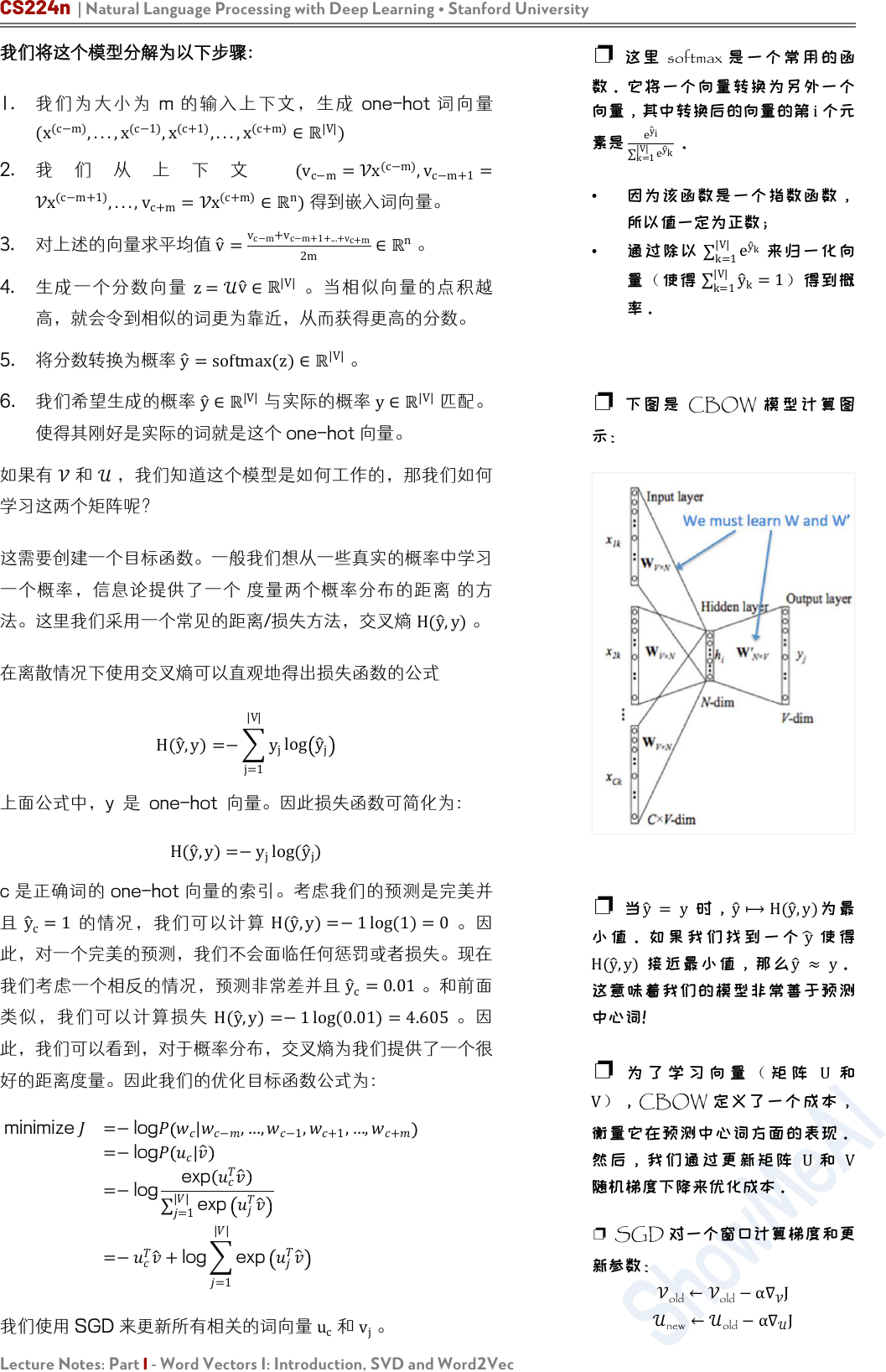CS224n | Natural Language Processing with Deep Learning Stanford University
Lecture Notes: Part I - Word Vectors I: Introduction, SVD and Word2Vec

1. m one-hot
(x
(c−m)
,...,x
(c−1)
,x
(c+1)
,...,x
(c+m)
|V|
)
2.
(v
c−m
=x
(c−m)
,v
c−m+1
=
x
(c−m+1)
,...,v
c+m
=x
(c+m)
n
)得到嵌入词向量。
3. 对上述的向量求平均值 v
=
v
c−m
+v
c−m+1+...+v
c+m
2m
n
4. z=
v
|V|

5. 将分数转换为概率 y
=softmax(z)
|V|
6. 我们生成概率 y
|V|

|V|

，我道这

H(y
,y)

H(
y
,y)=
j=1
|V|
y
j
log y
j

H(
y
,y)=y
j
log(
y
j
)
c one-hot
y
c
=1
H(y
,y)=1 log(1)=0

y
c
=0.01
。和
H(y
,y)=1 log(0.01)=4.605

minimize =−log
|
−
,…,
−1
,
+1
,,
+
=−log
|
=−log
exp
=1
||
exp
=−
+
log
=1
||
exp

c
v
j
softmax

i

e
y
i
k=1
|V|
e
y
k

k=1
|V|
e
y
k

k=1
|V|
y
k
=1

CBOW

y
= y
y
H(y
,y)
y

H(y
,y)
y
y

U
V
CBOW
U
V

SGD 一个度和

old
old
α
J
new
old
α
J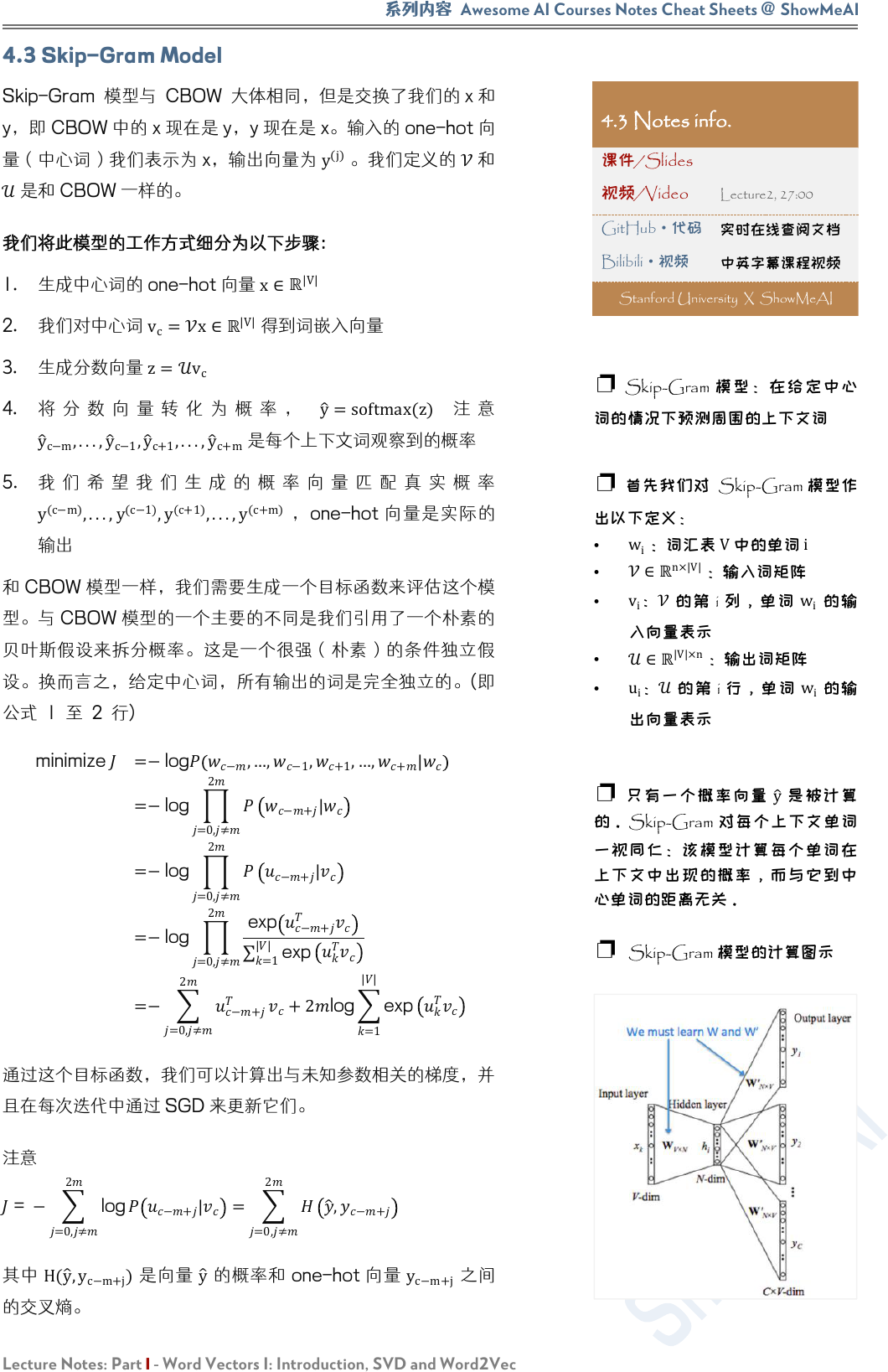Awesome AI Courses Notes Cheat Sheets
@
ShowMeAI
Lecture Notes: Part I - Word Vectors I: Introduction, SVD and Word2Vec
4.3 Skip-Gram Model
Skip-Gram 模型与 CBOW 大体相同，但是交换了我们 x
y，即 CBOW x 现在 yy 现在 x。输 one-hot

y
(j)
。我们定

1. 生成中心词的 one-hot 向量 x
|V|
2. 我们对中心词
v
c
=x
|V|

3. 生成分数向量
z=v
c
4. y
=softmax(z)
y
c−m
,...,y
c−1
,y
c+1
,...,y
c+m

5.
y
(c−m)
,...,y
(c−1)
,y
(c+1)
,...,y
(c+m)
one-hot

CBOW 型一，我需要生成目标数来估这

(

minimize
=−log
−
,…,
−1
,
+1
,…,
+
|
=−log
=0,≠
2
−+
|
=−log
=0,≠
2
−+
|
=−log
=0,≠
2
exp
−+
=1
||
exp
=−
=0,≠
2
−+
+2log
=1
||
exp

=
=0,≠
2
log
−+
|
=
=0,≠
2
,
−+
H(
y
,
y
c−m+j
)
y
one-hot y
c−m+j

4.3 Notes info.

GitHub·代码
Bilibili
·视频
Stanford University X ShowMeAI
Skip-Gram

w
i
：词汇表
V

i
n×|V|
：输入词矩
v
i
i
w
i

|V|×n
：输出词矩
u
i
i
w
i

y
Skip-Gram

Skip-Gram 模型的计算图CS224n | Natural Language Processing with Deep Learning Stanford University
Lecture Notes: Part I - Word Vectors I: Introduction, SVD and Word2Vec
4.4 Negative Sampling
|V|
O(|V|)

P
n
(w)

Mikolov Distributed Representations of Words and
Phrases and their Compositionality.中提

P(D=1∣w,c) (w,c)

P(D=1∣w,c)
sigmoid 函数建模：
(=1|,,)=
=
1
1+
−

P(D=1∣w,c)
P(D=0∣w,c)

θ

= argmax
(,)∈
(=1|,,)
(,)∈
(=0|,,)
= argmax
(,)∈
(=1|,,)
(,)∈
( 1(=1|,,))
= argmax
(,)∈
log (=1|,,)+
(,)∈
log (1(=1|,,))
=argmax
(,)∈
log
1
1+
exp
+
(,)∈
log
1
1
1+exp
=argmax
(,)∈
log
1
1+exp
+
(,)∈
log
1
1+
exp
4.4 Notes info.

GitHub
·代码
Bilibili·视频
Stanford University X ShowMeAI
softmax
CBOW Skip
Gram J

sigmoid softmax 1D

()=
1
1+
−
Sigmoid 函数Awesome AI Courses Notes Cheat Sheets
@
ShowMeAI
Lecture Notes: Part I - Word Vectors I: Introduction, SVD and Word2Vec

=−
(,)∈
log
1
1+exp
(,)∈
log
1
1+exp
D
“stock boil fish is toy”

D

c

cm+j的新目标函数为：
−
−+
=1
log
CBOW
v
=
v
c−m
+v
c−m+1
+...+v
c+m
2m

c

−log σ(u
c
T
v
)
k=1
K
l og σ(u
k
T
v
)

{
u
k
∣k=1...K}

P
n
(w)有很
3/4 Unigram 3/4

:0.9
3/4
= 0.92
:0.09
3/4
= 0.16
:0.01
3/4
= 0.032
“Bombastic“is”

4.5 Hierarchical Softmax
Mikolov Distributed Representations of Words and
Phrases and their Compositionality. hierarchical
softmax softmax

w
i
w
p(w∣w
i
) w

O(log(|V|))
，对应着路径的长度。

−+
+log
=1
||
exp
u
CBOW softmax

u
c
T
v
+log
j=1
|V|
exp(
u
j
T
v
)
Hierarchical softmax 使
O(log(|V|))

O(|V|)CS224n | Natural Language Processing with Deep Learning Stanford University
Lecture Notes: Part I - Word Vectors I: Introduction, SVD and Word2Vec

L(w
2
)
3
n(w,i)
v
n(w,i)
i n(w,1)
n(w,L(w)) w
n
ch(n)

|
=
=1
()−1
[(,+1)=ch((,))]
(,)

[]=
1 if is true
−1 otherwise

[n(w,j+1)=ch(n(w,j))]
1

[n(w,j+1)=ch(n(w,j))]
n

v
n
T
v
w
i

+
=1

w=1
|V|
P
(w∣w
i
)=1
，和 softmax

v
w
i
v
n(w,j)
T
w
2

2

2
|
=
2
,1
,left
2
,2
,left
2
,3 ,
right
=
2
,1
2
,2
2
,3
−log P(w∣w
i
)

Mikolov Distributed Representations of Words and
Phrases and their Compositionality.中使

Hierarchical softmax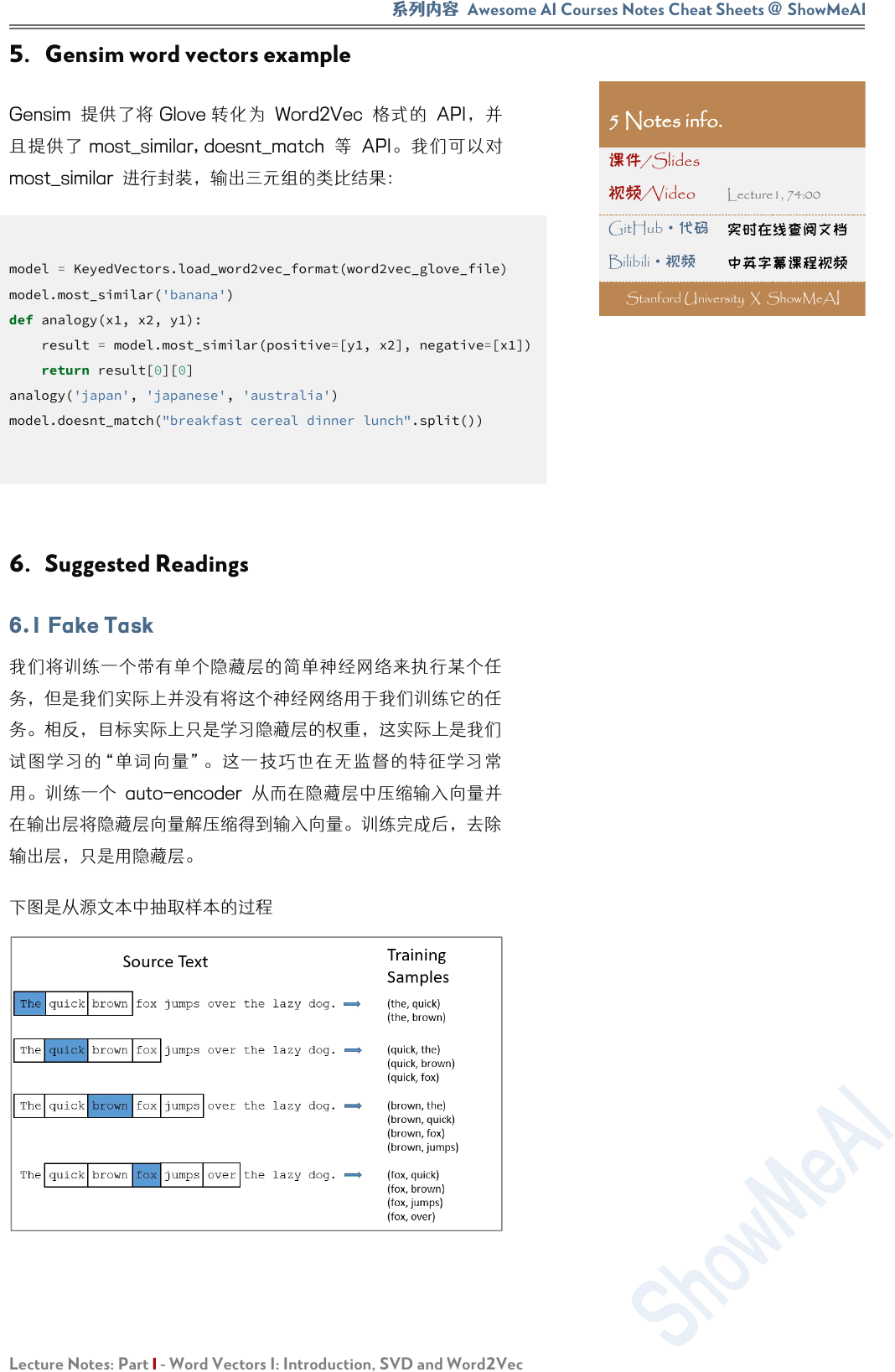Awesome AI Courses Notes Cheat Sheets
@
ShowMeAI
Lecture Notes: Part I - Word Vectors I: Introduction, SVD and Word2Vec
5. Gensim word vectors example
Gensim Glove Word2Vec API
most_similar, doesnt_match API
most_similar 进行封装，输出三元组的类比结果：
model.most_similar('banana')
def analogy(x1, x2, y1):
result = model.most_similar(positive=[y1, x2], negative=[x1])
return result
analogy('japan', 'japanese', 'australia')
model.doesnt_match("breakfast cereal dinner lunch".split())

5 Notes info.

GitHub·代码
Bilibili
·视频
Stanford University X ShowMeA
ICS224n | Natural Language Processing with Deep Learning Stanford University
Lecture Notes: Part I - Word Vectors I: Introduction, SVD and Word2Vec

Efficient Estimation of Word Representations in Vector
Space(original word2vec paper)
Distributed Representations of Words and Phrases and their
Compositionality (negative sampling paper)
Word2Vec Tutorial - The Skip-Gram Model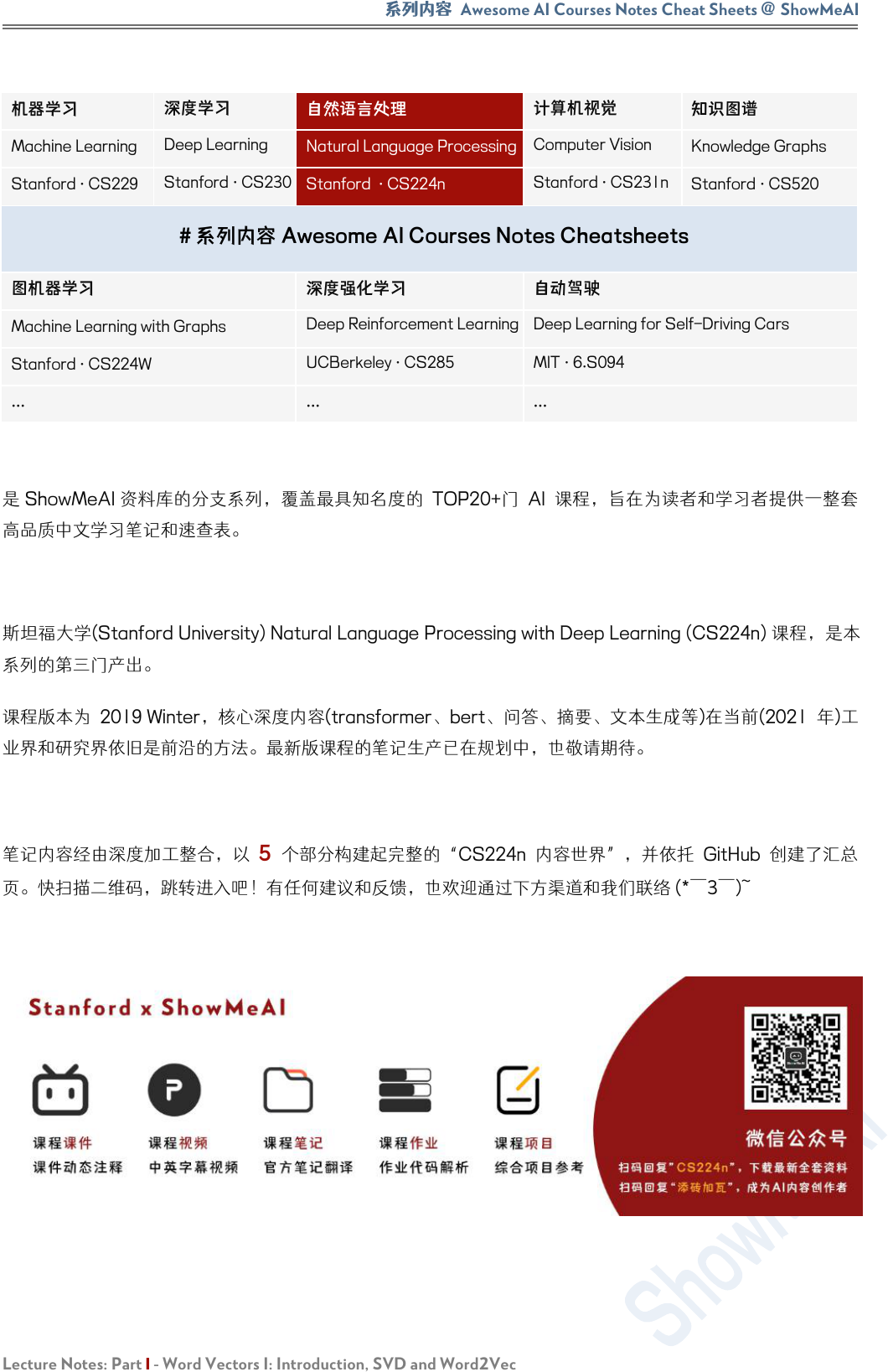Awesome AI Courses Notes Cheat Sheets
@
ShowMeAI
Lecture Notes: Part I - Word Vectors I: Introduction, SVD and Word2Vec

Machine Learning
Deep Learning
Natural Language Processing
Computer Vision
Knowledge Graphs
Stanford · CS229
Stanford · CS230
Stanford · CS224n
Stanford · CS231n
Stanford · CS520
# 系列内容 Awesome AI Courses Notes Cheatsheets

Machine Learning with Graphs
Deep Reinforcement Learning
Deep Learning for Self-Driving Cars
Stanford · CS224W
UCBerkeley · CS285
MIT · 6.S094
...
...
...
ShowMeAI 的分，覆具知TOP20+ AI 旨在和学供一